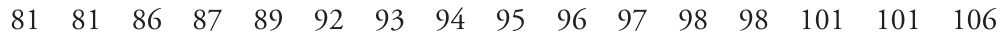# Quiz 5

Date: 02/21/2020

## Instructions:

Write your name in the top-right corner of the page. You are not allowed any resources other than a standard scientific, non-graphing calculator.

## Problems:

### Problem 1:

#### 1.1 (2 points):

Suppose we have the following samples: $$\{21, 22, 23\}$$. Compute the standard deviation of these samples.

#### 1.2 (2 points):

Suppose we have the following samples: $$\{1, 1.5, 2\}$$. Compute the standard deviation of these samples.

#### 1.3 (1 point):

Use the coefficient of variation to explain which of the two sample data sets in parts 1.1 and 1.2 has a larger relative variation.

### Problem 2 (5 points):

Below are the interval times (minutes) between eruptions of the Old Faithful geyser in Yellowstone National Park (based on data from the U.S. National Park Service). Compute the five number summary of this data.